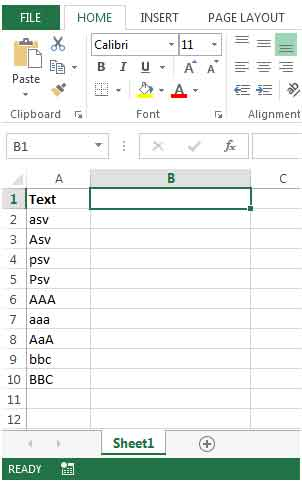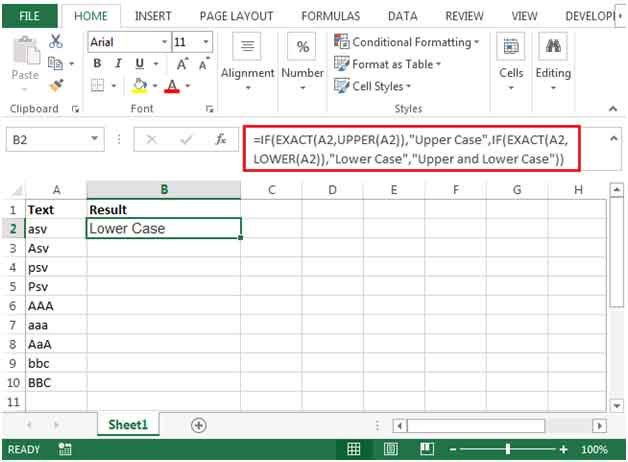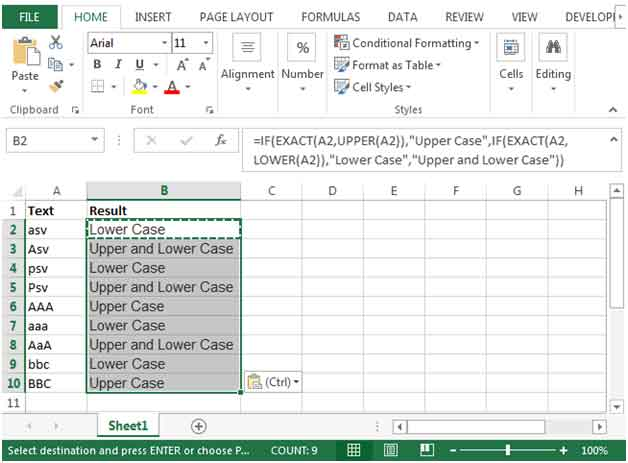# How to Identify Lower Upper Case Letters in Excel 2010

While working on importing data from the internet or softwares like ACCESS, SQL, etc, there could be a need to update the text to proper case.

Excel provides built-in functions for users to change the case of the text to upper, proper & lower cases.

We will use the IF, UPPER, LOWER & EXACT functions together to make a formula that will work in a single cell taking a reference from the corresponding cell.

IF FUNCTION: The IF function checks if the condition you specify is TRUE or FALSE. If the function evaluates to true, it returns one value, else it returns the 2nd value given in the function.

Syntax = IF(logical_test,value_if_true,value_if_false)

logical_test: Logical test is the condition or criteria to be tested.

value_if_true: The value that should be returned if the logical_testreturns TRUE.

value_if_false: The value that should be returned if the logical_testreturns FALSE.

UPPER FUNCTION – The Upper function will convert a lower or proper case string into UPPER case letters. For example: abc or Abc to ABC.

Syntax =UPPER(text)

text: Itis the text which should be converted to uppercase.

LOWER FUNCTION – The Lower function will convert UPPER or PROPER case string into LOWER case letters. For example, ABC or Abc to abc.

Syntax =LOWER(text)

text: It is the text which should be converted to lowercase.

EXACT FUNCTION – The Exact function checks whether two text strings are exactly the same, and returns TRUE or FALSE. EXACT is case-sensitive.

Syntax =EXACT(text1,text2)

text1: Itis the first text string to be compared.

text2: It is the second text string to be compared.

Let us take an example:

• We have some text in column A which is displayed in various cases – upper, proper, lower, etc.• Enter the formula in cell B2

=IF(EXACT(A2,UPPER(A2)),"Upper Case",IF(EXACT(A2,LOWER(A2)),"Lower Case","Upper and Lower Case"))• The Exact function is used to determine whether the value returned is TRUE or FALSE.
• The value returned by the Exact function will then be passed on to the IF function.
• The IF function will treat this value as its first argument. If the value returns a TRUE then “Upper Case” will be the output.  If the value returns a FALSE then another IF function will check if the value in the cell contains any lower case characters. If it’s found to be TRUE then “Lower Case” will be the output, but if its found to be FALSE then the output will be “Upper and Lower Case”
• By copying the formula to the below range, we will get the desired output.1.How to search for CAPITAL letter A in a word like " fistA" . I want to give filtering to list all the words Containing Capital Letter A in list of 100 words.. is it possible?

•You can use function like FIND. It is case sensitive and can help you find the location of a specific text.
=FIND("A","fistA")
This formula will return 5. it mean it contains A. if it doesn't have A then it would return #VALUE! error. Using this you can filter your data. Here is the link of find function.
https://www.exceltip.com/lookup-formulas/excel-find-function.html

2.Can't get this to work. The routine continues to return "#NAME?"

FILTER FLUID #NAME?
Valve, Fuel Control #NAME?
PUMP FUEL ELECTRICAL #NAME?
Pump,Fuel,Metering And Distribution #NAME?
FILTER ELEMENT AIR CONDITIONING #NAME?
EVAPORATOR COIL,REFRIGERATION #NAME?
PAN TRUCK #NAME?
COVER AIR CONDITIONER #NAME?
EVAPORATOR AIR CONDITIONER #NAME?
CONDENSER REFRIGERATION #NAME?
SHACKLE #NAME?
Latch,Safety,Hook #NAME?
TERMINAL,ROPE,SWAGING #NAME?
Hook,Chain,S #NAME?
Hook,Hoist #NAME?
Clamp,Wire Rope,Saddled,used for 5 ton tanker trailer model M967A2 and M969A3,accommodated material diameter .188 IN #NAME?

•Hi, here is the correct formula:

=IF(EXACT(A2,UPPER(A2)),"Upper Case",IF(EXACT(A2,LOWER(A2)),"Lower Case","Upper and Lower Case"))

•I see when i paste the formula the quotation marks is different than in my excel, so just correct that and it will work

" " and not ” ”

•The formula works but web pages do strange things to quote marks - copy and paste it into your excel then replace the quote marks with straight quotes and it works.

Terms and Conditions of use

The applications/code on this site are distributed as is and without warranties or liability. In no event shall the owner of the copyrights, or the authors of the applications/code be liable for any loss of profit, any problems or any damage resulting from the use or evaluation of the applications/code.